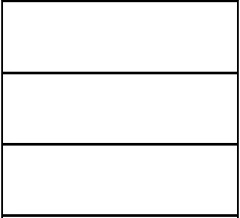# Circumference 6598

Adam had three identical rectangles. He put them together and got a rectangle with a circumference of 50 cm. Then he placed them differently and got a rectangle with a larger circumference. Calculate its perimeter.

o2 =  70 cm

### Step-by-step explanation:Did you find an error or inaccuracy? Feel free to write us. Thank you!

Tips for related online calculators
Do you have a linear equation or system of equations and looking for its solution? Or do you have a quadratic equation?

#### Grade of the word problem:

We encourage you to watch this tutorial video on this math problem: